# COUNTA Function – Examples in Excel, VBA, Google Sheets

This tutorial demonstrates how to use the Excel COUNTA Function in Excel to count non-blank numbers.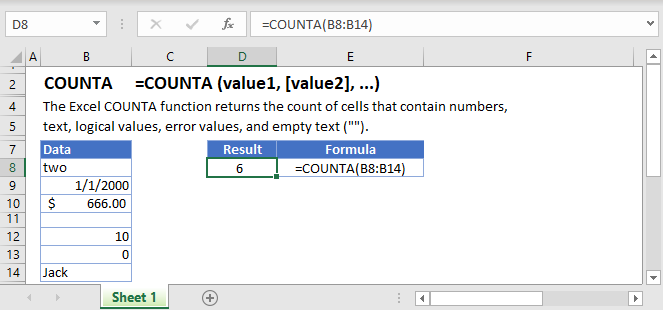## COUNTA Function Overview

The COUNTA Function Counts the number of non-blank cells within a series

To use the COUNTA Excel Worksheet Function, select a cell and type: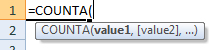(Notice how the formula inputs appear)

## COUNTA function Syntax and inputs:

The Syntax for the COUNTA Formula is:

`=COUNTA(value1,[value2],...)`

array – An array of numbers, text, or blank values.

# What is the COUNTA Function?

The Excel COUNTA Function returns the number of cells within a given range that are not empty.

## How to Use the COUNTA Function

Use the Excel COUNTA Function as shown below:

`=COUNTA(B3:B11)`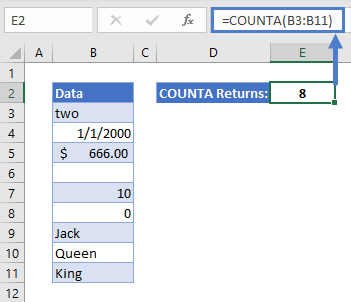In this example, COUNTA returns 8, since there is only one empty cell in the range B3:B11, and eight that are not empty.

## COUNTA Counts Empty Strings

Note that COUNTA counts cells with any kind of data in them – even if it is not visible to the user. See the example below: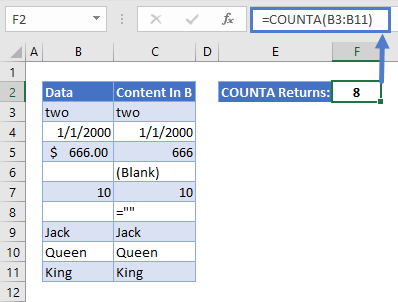It looks like there are seven non-empty cells in this range, so why has COUNTA returned 8?

It’s because cell B8 is not actually empty. It contains the formula for an empty string:

=””

Because of this, COUNTA counts that cell.

## How to Count Cells that Are Empty

What if you want to count the number of cells in a range that ARE empty? We can do that too! Keeping the same example as above, we could just use the following formula:

`=(ROWS(B3:B11)*COLUMNS(B3:B11)) - COUNTA(B3:B11)`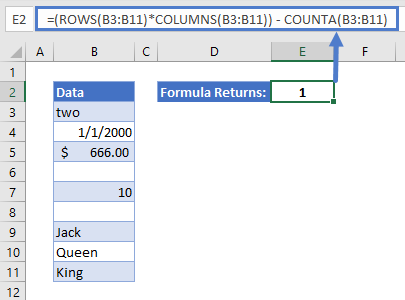In this formula:

• ROWS returns the number of rows within a range (9 in this case)
• COLUMNS returns the number of columns within a range (just 1 here)
• Multiplying ROWS and COLUMNS together returns the total number of cells in that range (9 * 1 = 9)
• Now we just need to subtract the number of non-empty cells from the total, and we use COUNTA for that (9 – 8 = 1)

Again, since B8 contains =”” it is not counted as empty. Only B6 is truly empty, so the formula returns 1.

If you need to count the number of empty cells but you want to include cells that contain information but no visible data (like empty strings), use COUNTBLANK instead <<link>>.

The COUNTA Function works exactly the same in Google Sheets as in Excel: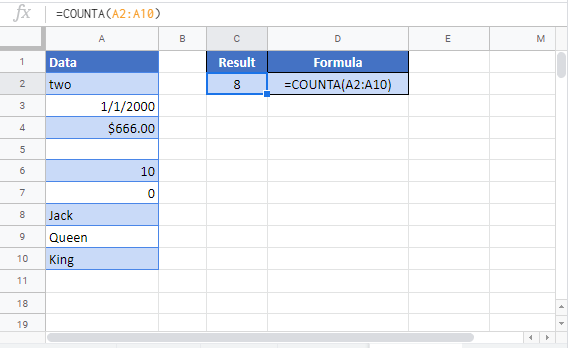## COUNTA Examples in VBA

You can also use the COUNTA function in VBA. Type:
`application.worksheetfunction.counta(value1,value2)`
For the function arguments (array, etc.), you can either enter them directly into the function, or define variables to use instead.

To count the non-blank cells in the following range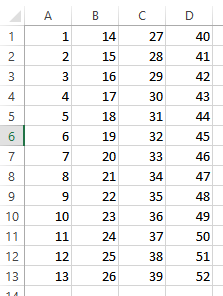we would execute the following VBA code

```Private Sub Excel_VBAExample_CountA()
Dim CountValues  As Variant
CountValues = Application.WorksheetFunction.CountA(Range("A1:D13"))
MsgBox ("CountA result is: " & CountValues)
End Sub```

The result would be :

`CountA result is: 52`

We can count the non-blank cells for up to 30 ranges defined. If we need to count  non-blank cells for the following :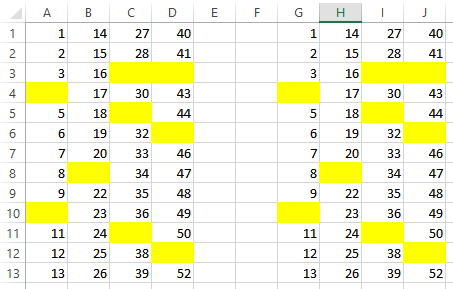Executing the code below:

`CountValues=Application.WorksheetFunction.CountA(Range("A1:D13"),Range("G1:J13"))`

Would come up with:

`CountA result is: 86`

as there are 86 non-blank cells in these two ranges